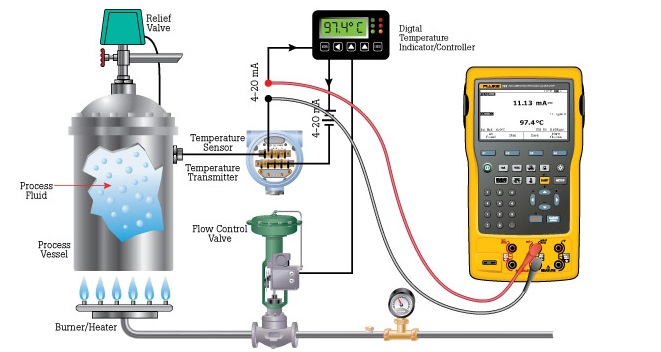# How to convert mA signal into % in instruments?(ie 4-20 mA=0 to100%)## Example calculation: controller output to valve

An electronic loop controller outputs a signal of 8.55 mA to a direct-responding control valve (where 4 mA is shut and 20 mA is wide open). How far open should the control valve be at this MV signal level?

We must convert the milliamp signal value into a percentage of valve travel. This means determining the percentage value of the 8.55 mA signal on the 4-20 mA range. First, we need to manipulate the percentage-milliamp formula to solve for percentage (x):Next, we plug in the 8.55 mA signal value and solve for x:Therefore, the control valve should be 28.4 % open when the MV signal is at a value of 8.55 mA.

## Example calculation: flow transmitter

A flow transmitter is ranged 0 to 350 gallons per minute, 4-20 mA output, direct-responding. Calculate the current signal value at a flow rate of 204 GPM.

First, we convert the flow value of 204 GPM into a percentage of range. This is a simple matter of division, since the flow measurement range is zero-based:Next, we take this percentage value and translate it into a milliamp value using the formula previously shown:Therefore, the transmitter should output a PV signal of 13.3 mA at a flow rate of 204 GPM.

## Example calculation: temperature transmitter

An electronic temperature transmitter is ranged 50 to 140 degrees Fahrenheit and has a 4-20 mA output signal. Calculate the current output by this transmitter if the measured temperature is 79 degrees Fahrenheit.

First, we convert the temperature value of 79 degrees into a percentage of range based on the knowledge of the temperature range span (140 degrees − 50 degrees = 90 degrees) and lower-range value (LRV = 50 degrees). We may do so by manipulating the general formula for any linear measurement to solve for x:Next, we take this percentage value and translate it into a 4-20 mA current value using the formula previously shown:Therefore, the transmitter should output a PV signal of 9.16 at a temperature of 79o F.

## Example calculation: pH transmitter

A pH transmitter has a calibrated range of 4 pH to 10 pH, with a 4-20 mA output signal. Calculate the pH sensed by the transmitter if its output signal is 11.3 mA.

First, we must convert the milliamp value into a percentage. Following the same technique we used for the control valve problem:Next, we take this percentage value and translate it into a pH value, given the transmitter’s measurement span of 6 pH (10 pH − 4 pH) and offset of 4 pH:Therefore, the transmitter’s 11.3 mA output signal reflects a measured pH value of 6.74 pH.

## Example calculation: reverse-acting I/P transducer signal

A current-to-pressure transducer is used to convert a 4-20 mA electronic signal into a 3-15 PSI pneumatic signal. This particular transducer is configured for reverse action instead of direct, meaning that its pressure output at 4 mA should be 15 PSI and its pressure output at 20 mA should be 3 PSI. Calculate the necessary current signal value to produce an output pressure of 12.7 PSI. Reverse-acting instruments are still linear, and therefore still follow the slope-intercept line formula y = mx + b. The only differences are a negative slope and a different intercept value. Instead of y = 16x + 4 as is the case for direct-acting instruments, this reverse-acting instrument follows the linear equation y = −16x + 20:First, we need to to convert the pressure signal value of 12.7 PSI into a percentage of 3-15 PSI range. We will manipulate the percentage-pressure formula to solve for x:Next, we plug in the 12.7 PSI signal value and solve for x:This tells us that 12.7 PSI represents 80.8 % of the 3-15 PSI signal range. Plugging this percentage value into our modified (negative-slope) percentage-current formula will tell us how much current is necessary to generate this 12.7 PSI pneumatic output:Therefore, a current signal of 7.07 mA is necessary to drive the output of this reverse-acting I/P transducer to a pressure of 12.7 PSI.